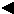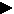Using Fundamental Fiber Properties Data and Stelometer Data Obtained at Each of Four Gauge Lengths to Analyze, Interpret, and Predict Yarn Data. Part I. Method of Determining the Actual Gauge Length of the Nominal Zero Gauge Length Used for Cotton L. B. De Luca and D. P. Thibodeaux ABSTRACT Stelometer data on forty-three different cottons were measured at 0, 1, 2, and 3.2 mm gauge lengths. Bundle breaking load as a linear function of bundle weight and bundle breaking extension, respectively, were used to interpolate the bundle weight and bundle breaking extension to 5 kilograms breaking load for each cotton. The normalized values of bundle tenacity and breaking extension were used to determine, theoretically, a true gauge length in place of 'nominal zero gauge length' for each cotton by simultaneous solutions of the equations: (1) (Bundle Extension)(GL) = A (.) (Gauge Length) + C (2) (Bundle Tenacity)(GL) = C . (Bundle Extension)(GL) + D Gauge Length where: A,B,C, and D are constants. These methods permit bundle elongation and secant modulus to be determined for the nominal zero gauge length data and allow comparisons to be made with similar bundle data obtained at 1, 2, and 3.2 mm gauge lengths. Preliminary multiple correlation analysis of bundle data at 3.2 mm (one-eighth inch) gauge length and select fiber property data with yarn data are made and compared with extant data at one-eighth inch gauge length to demonstrate the method of analysis.[Previous] [Next]